One common programming question is how to randomly shuffle an array of numbers in-place. There are a few wrong answers to this question - some simple shuffles people tend to think of immediately turn out to be inadequate. In particular, the most common naive algorithm that comes up is :

```naive_shuffle(arr):
if len(arr) > 1:
for i in 0 .. len(arr) - 1:
s = random from inclusive range [0:len(arr)-1]
swap arr[s] with arr[i]
```

This algorithm produces results that are badly skewed. For more information consult this post by Jeff Attwood, and this SO discussion.

The correct answer is to use the Fisher-Yates shuffle algorithm:

```fisher_yates_shuffle(arr):
if len(arr) > 1:
i = len(arr) - 1
while i > 0:
s = random from inclusive range [0:i]
swap arr[s] with arr[i]
i--
```

It was first invented as a paper-and-pencil method back in 1938, and later was popularized by Donald Knuth in Volume II of TAOCP. For this reason it's also sometimes called the Fisher-Yates-Knuth algorithm. In this article I don't aim to compare Fisher-Yates to the naive algorithm. Nor do I plan to explain why the naive shuffle doesn't work. Others have done it before me, see the references to Jeff's post and the SO discussion above.

What I do plan to do, however, is to explain why the Fisher-Yates algorithm works. To put it more formally, why given a good random-number generator, the Fisher-Yates shuffle produces a uniform shuffle of an array in which every permutation is equally likely. And my plan is not to prove the shuffle's correctness mathematically, but rather to explain it intuitively. I personally find it much simpler to remember an algorithm once I understand the intuition behind it.

### An analogy

Imagine a magician's hat:And a bunch of distinct balls. Let's take pool balls for the example:Suppose you place all those balls into the hat  and stir them really well. Now, you look away and start taking balls randomly out of the hat and placing them in a line. Assuming the hat stir was random and you can't distinguish the balls by touch alone, once the hat is empty, the resulting line is a random permutation of the balls. No ball had a larger chance of being the first in line than any other ball. After that, all the remaining balls in the hat had an equal chance of being the second in line, and so on. Again, this isn't a rigorous proof, but the point of this article is intuition.

If you understand why this procedure produces a random shuffle of the balls, you can understand Fisher-Yates, because it is just a variation on the same theme.

### The intuition behind Fisher-Yates shuffling

The Fisher-Yates shuffle performs a procedure similar to pulling balls at random from a hat. Here's the algorithm once again, this time in my favorite pseudo-code format, Python :

```def fisher_yates(arr):
if len(arr) > 1:
i = len(arr) - 1
while i > 0:
s = randint(0, i)
arr[i], arr[s] = arr[s], arr[i]
i -= 1
```

The trick is doing it in-place with no extra memory. The following illustration step by step should explain what's going on. Let's start with an array of 4 elements: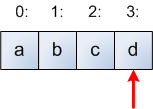The array contains the letters a, b, c, d at indices [0:3]. The red arrow shows where i points initially. Now, the initial step in the loop picks a random index in the range [0:i], which is [0:3] in the first iteration. Suppose the index 1 was picked, and the code swaps element 1 with element 3 (which is the initial i). So after the first iteration the array looks like this: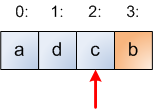Notice that I colored the part of the array to the right of i in another color. Here's spoiler: The blue part of the array is the hat, and the orange part is the line where the random permutation is being built. Let's make one more step of the loop. A random number in the range [0:2] has to be picked, so suppose 2 is picked. Therefore, the swap just leaves the element at index 2 in its original place: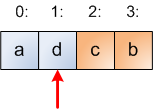We make one more step. Suppose 0 is picked at random from [0:1] so elements at indices 0 and 1 are swapped: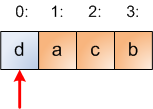At this point we're done. There's only one ball left in the hat, so it will be surely picked next. This is why the loop of the algorithm runs while i > 0 - once i reaches 0, the algorithm finishes: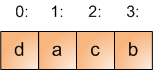So, to understand why the Fisher-Yates shuffling algorithm works, keep in mind the following: the algorithm makes a "virtual" division of the array it shuffles into two parts. The part at indices [0:i] is the hat, from which elements will be picked at random. The part to the right of i (that is, [i+1:len(arr)-1]) is the final line where the random permutation is being formed. In each step of the algorithm, it picks one element from the hat and adds it to the line, removing it from the hat.

Some final notes:

• Since all the indices [0:i] are in the hat, the selection can pick i itself. In such case there's no real swapping being done, but the element at index i moves from the hat and to the line. Having the selection from range [0:i] is crucial to the correctness of the algorithm. A common implementation mistake is to make this range [0:i-1], which causes the shuffle to be non-uniform.
• The vast majority of implementations you'll see online run the algorithm from the end of the array down. But this isn't set in stone - it's just a convention. The algorithm will work equally well with i starting at 0 and running until the end of the array, picking items in the range [i:len(arr)-1] at each step.

### Conclusion

Random shuffling is important for many applications. Although it's a seemingly simple operation, it's easy to do wrong. The Internet is abound with stories of gambling companies losing money because their shuffles weren't random enough.

The Fisher-Yates algorithm produces a uniform shuffling of an array. It's optimally efficient both in runtime (running in O(len(arr))) and space (the shuffle is done in-place, using only O(1) extra memory).

In this article I aimed to explain the intuition behind the algorithm, firmly believing that a real, deep understanding of something  is both intellectually rewarding and useful.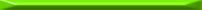Here, like in the rest of the article, assume that all arrays are 0-based, i.e. their first element is at index 0.
  Yes, I know it will become heavy. Actually, if you've noticed it you probably have a slight case of ADHD. Stay focused on the algorithm, OK? If you still can't, imagine that these are mini-pool balls.
  This implementation is very similar to the code of random.shuffle from the standard library.
  In other words, grokking it.